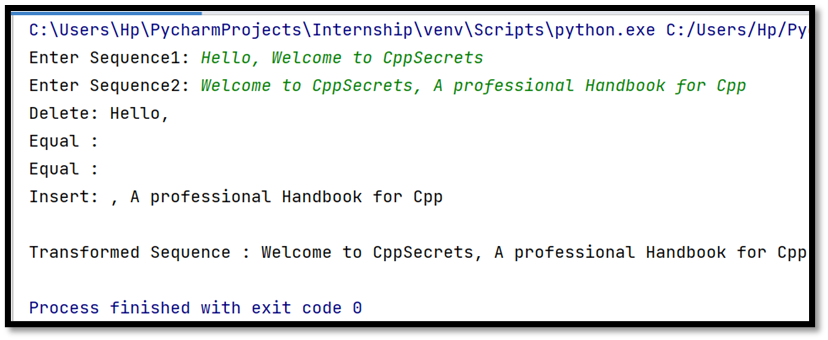# get_grouped_opcodes()

get_grouped_opcodes()
is  a method of
Sequence matcher class of
difflib module. Like the get_opcodes() method this also specifies how to
transform first sequence(a) into Second sequence(b).

This method returns a generator instance where the individual element is a list of groups
specifying operations to be performed to transform the  sequence .

It does that with n lines of context.Starting
with the groups returned by
get_opcodes(), this method splits out smaller
change clusters and eliminates intervening ranges which have no changes.

The groups are returned in the same format as get_opcodes().

Tag: is an element specifying one of the operations that should be performed on the first
sequence to transform it to second.

[i1, i2,j1, j2]:  Are 2 indices from the first
sequence and 2 indices from the second sequence specifying where operations
should be applied. The first tuple has i1 == j1 == 0, and remaining tuples have
i1 equal to the i2 from the preceding tuple, and, likewise, j1 equal to the
previous j2.

The tag values are strings, with the following meanings

1. Replace: It specifies that a[i1:i2] should be replaced by b[j1:j2].
2. Delete - It specifies that a[i1:i2] should be deleted.
3. Insert - It b[j1:j2] should be inserted at a[i1].
4. Equal - It means that a[i1:i2] and b[j1:j2] are same.

SYNTAX

`  get_grouped_opcodes(n)`

Lets take an example:

CODE

`import difflibfrom difflib import SequenceMatcherString1 = input("Enter Sequence1: ")String2 = input("Enter Sequence2: ")Sequence = difflib.SequenceMatcher(a=String1, b=String2)String3 = list(String1)grouped_opcodes=Sequence.get_grouped_opcodes(n=0)for groups in grouped_opcodes:    for tag, i1,i2,j1,j2 in groups:        if tag == "delete":            print("Delete: {} ".format(String1[i1:i2]))            String3[i1:i2] = [""] * len(String3[i1:i2])        elif tag == "replace":            print("Replace: {}  ".format(String1[i1:i2], String2[j1:j2]))            String3[i1:i2] = String2[j1:j2]        elif tag == "insert":            print("Insert: {} ".format(String2[j1:j2], i1))            String3.insert(i1, String2[j1:j2])        elif tag == "equal":            print("Equal : {} ".format(String1[i1:i2]))print("\nTransformed Sequence : {}".format("".join(String3)))`

In the above code First we take two input sequences from the user which given to the
sequence matcher class. Then we create a third variable which is a list. This
list is a copy of the first list and has elements as a list of characters.
We then
loop through each group returned by get_grouped_opcodes() method and perform
operations specified in each group on the third sequence (which is a copy of
the first sequence) to transform it to the second sequence.

Here number of context lines is n=0

OUTPUT:Thus in this output the Equal Sequences are not printed